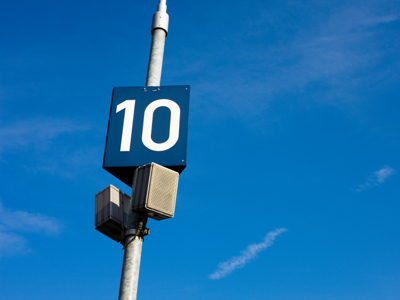10,000 ÷ 1,000 = 10.

# Multiplication and Division (Very Easy)

Division and multiplication is dividing and multiplying numbers. This is the first of our 11-plus Maths quizzes on multiplication and division. You should be able to do most of this stuff in your head. Knowing your times tables is always useful when it comes to multiplying and dividing.

You may be great at addition and subtraction, but not so good at multiplication and division. It's definitely worth spending some time practising these valuable skills. Simple multiplication and division are used by us near-enough every day. You don't want to find yourself out of pocket because you couldn't do an easy division calculation.

Always remember in tests and exams to read the questions carefully to make sure you have understood what is required. Try these quizzes and see how good you are.

1.
What is the correct answer to the given calculation?
3 doubled
9
6
27
81
'Double' means multiply by 2
2.
What is the correct answer to the given calculation?
85.60 ÷ 100
8.560
0.08560
0.8560
856
To divide 85.60 by 100 just move the decimal point 2 places to the left and put in a zero to show that there are no whole numbers: 85.60 ÷ 100 = 0.8560
3.
What is the correct answer to the given calculation?
10,000 × 100
10,000
100,000
1,000,000
10,000,000
To multiply 10,000 by 100, add together the number of zeros in the two numbers, then write 1 followed by the sum of the zeros: 10,000 × 100 = 1,000,000. Your final answer has six zeros because the sum of the zeros in the two numbers equals six. You should be able to do this in your head
4.
What is the correct answer to the given calculation?
10,000 ÷ 1,000
100
1,000
0.1
10
To divide 10,000 by 1,000, cancel as many zeros in 10,000 as there are in 1,000: 10,000 ÷ 1000 = 10. Your final answer has 1 zero because the 3 zeros in 10,000 cancelled with the 3 zeros in 1,000.You should be able to do this in your head
5.
What is the correct answer to the given calculation?
250 ÷ 10
0.25
2.5
0.025
25
To divide 250 by 10, just remove the last zero from the number and you are done: 250 ÷ 10 = 25. You should be able to do this in your head
6.
What is the correct answer to the given calculation?
8
4
16
32
7.
What is the correct answer to the given calculation?
1,000 × 4.5
45
450
4.5
4,500
To multiply 4.5 by 1,000, just move the decimal point 3 places to the right and add in a zero for each place position if there are no digits in those place positions in the number: 1,000 × 4.5 = 4,500. You should be able to do this in your head
8.
What is the correct answer to the given calculation?
300 × 12
3.6
3,600
36
360
To multiply 12 by 300, multiply the non zero numbers and just stick on two zeros at the end: 300 × 12 = 3 × 12 , and 36 + 00 = 3,600. You should be able to do this in your head
9.
What is the correct answer to the given calculation?
100 × 24
2,400
240
24
2.4
To multiply 24 by 100, multiply the non zero numbers and just stick on the two zeros at the end: 100 × 24 = 1 × 24 , and 24 + 00 = 2,400. You should be able to do this in your head
10.
What is the correct answer to the given calculation?
4 trebled
12
8
16
64
'Treble' means multiply by 3: triple = treble
Author:  Frank Evans